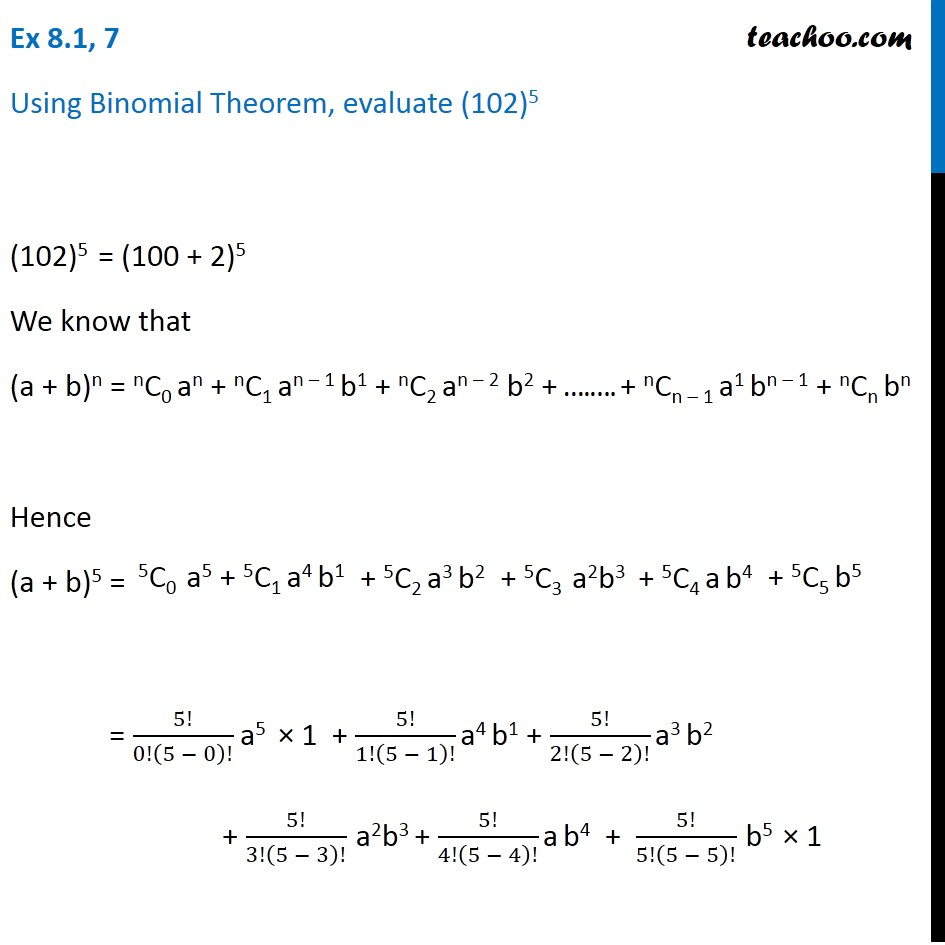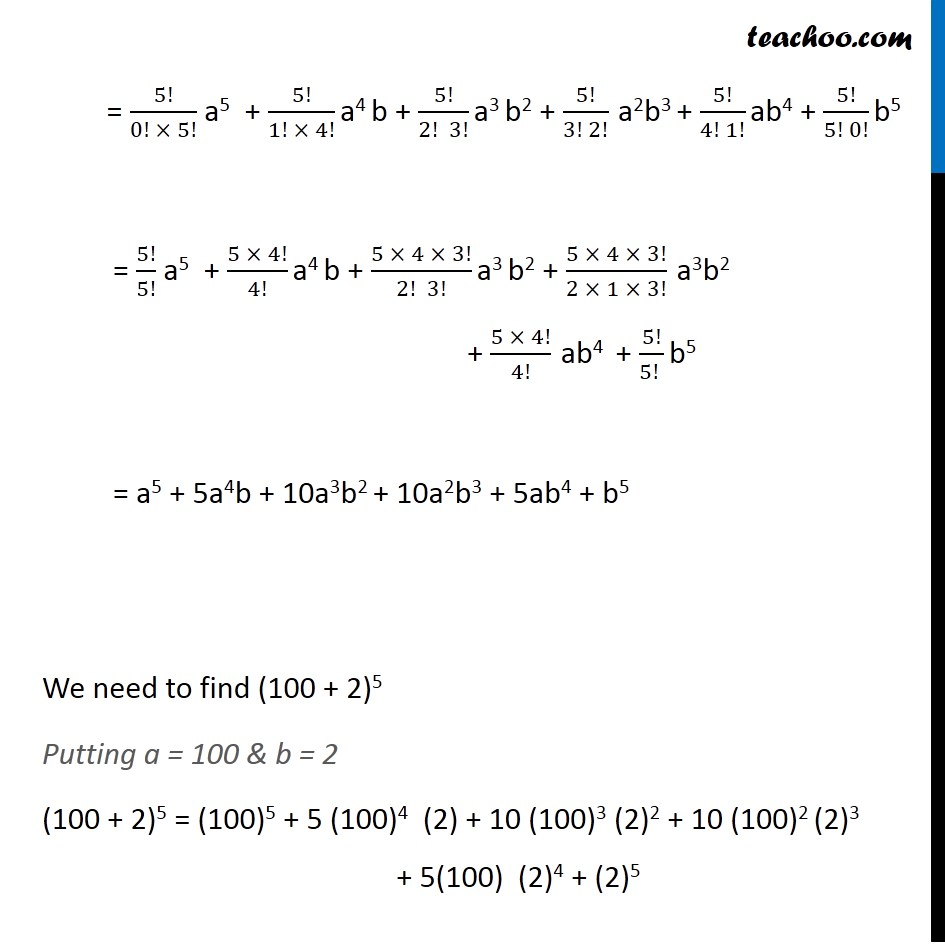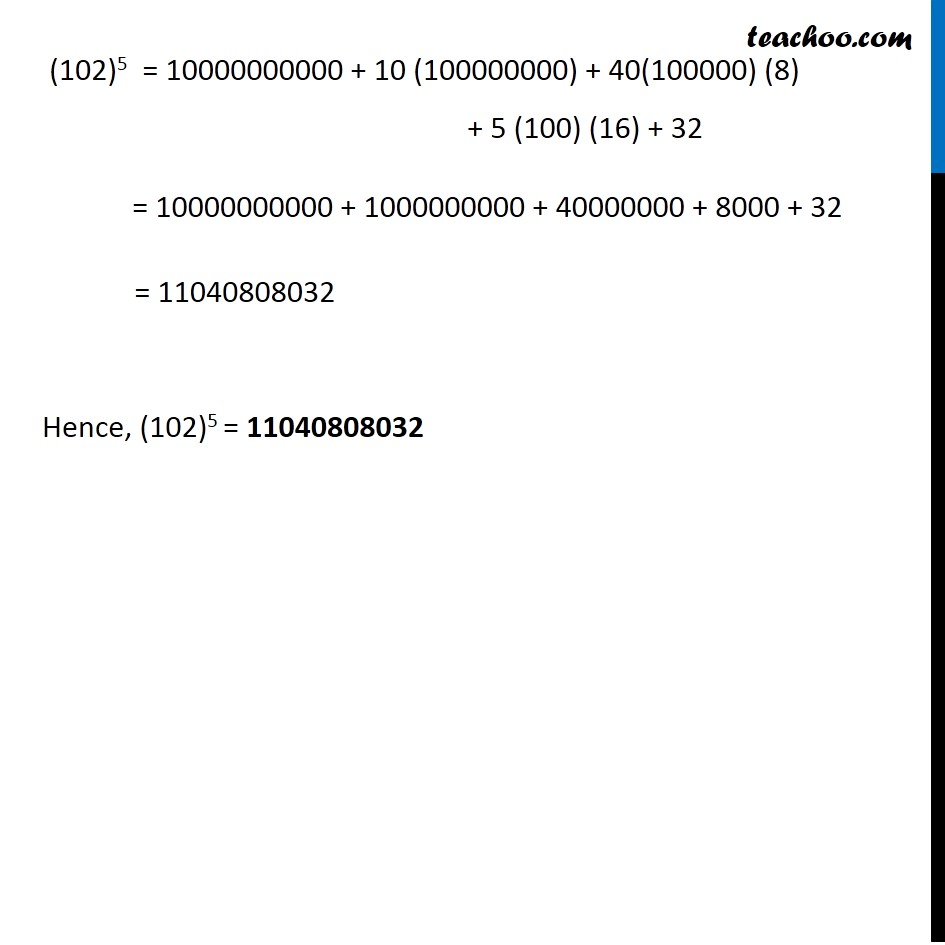Ex 8.1

Chapter 8 Class 11 Binomial Theorem (Deleted)
Serial order wise549 people joined Teachoo Black. What are you waiting for?

### Transcript

Ex 8.1, 7 Using Binomial Theorem, evaluate (102)5 (102)5 = (100 + 2)5 We know that (a + b)n = nC0 an + nC1 an – 1 b1 + nC2 an – 2 b2 + ….…. + nCn – 1 a1 bn – 1 + nCn bn Hence (a + b)5 = = 5!/0!(5 − 0)! a5 × 1 + 5!/1!(5 − 1)! a4 b1 + 5!/2!(5 − 2)! a3 b2 + 5!/3!(5 − 3)! a2b3 + 5!/4!(5 − 4)! a b4 + 5!/5!(5 − 5)! b5 × 1 = 5!/(0! × 5!) a5 + 5!/(1! × 4!) a4 b + 5!/(2! 3!) a3 b2 + 5!/(3! 2!) a2b3 + 5!/(4! 1!) ab4 + 5!/(5! 0!) b5 = 5!/5! a5 + (5 × 4!)/4! a4 b + (5 × 4 × 3!)/(2! 3!) a3 b2 + (5 × 4 × 3!)/(2 × 1 × 3!) a3b2 + (5 × 4!)/4! ab4 + 5!/(5! ) b5 = a5 + 5a4b + 10a3b2 + 10a2b3 + 5ab4 + b5 We need to find (100 + 2)5 Putting a = 100 & b = 2 (100 + 2)5 = (100)5 + 5 (100)4 (2) + 10 (100)3 (2)2 + 10 (100)2 (2)3 + 5(100) (2)4 + (2)5 (102)5 = 10000000000 + 10 (100000000) + 40(100000) (8) + 5 (100) (16) + 32 = 10000000000 + 1000000000 + 40000000 + 8000 + 32 = 11040808032 Hence, (102)5 = 11040808032